Quick start with R: Generalised Linear Model on binary data (Part 16)

In Part 15, we saw how to create a simple Generalised Linear Model on binary data using the glm() command. We continue with the same glm (modelling the vs variable on the weight and engine displacement) on the same data set – the mtcars dataset – which is built into R.
model <- glm(formula = vs ~ wt + disp, data = mtcars, family = binomial) summary(model)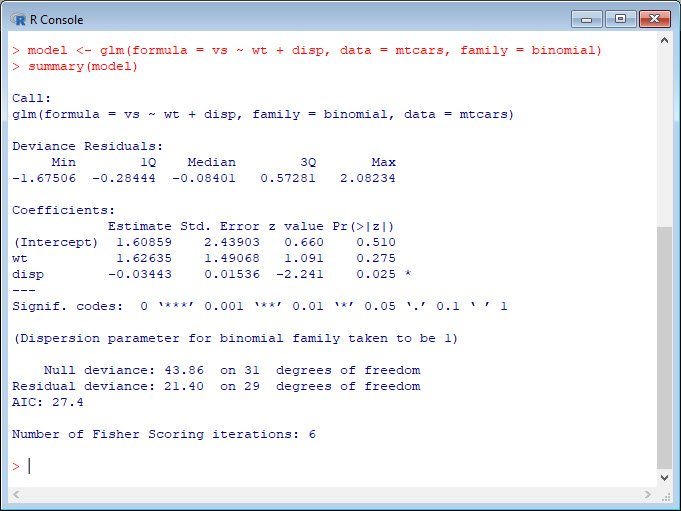We see that weight influences vs positively, while displacement has a slightly negative effect. We also see that the coefficient of weight is non-significant (p-value < 0.05) while the coefficient of displacement is significant. Later we will see how to investigate ways of improving our model.
However, the estimates (coefficients of the predictors weight and displacement) are now in units called logits. We will define the logit in a later blog.
We see the word Deviance twice over in the model output. Deviance is a measure of goodness of fit. R reports two forms of deviance – the null deviance and the residual deviance. The null deviance shows how well the response variable is predicted by a model that includes only the intercept (grand mean). For our example, we have a Chi square value of 43.9 on 31 degrees of freedom. This value indicates little fit (a significant difference between fitted values and observed values). Including the independent variables (disp and weight) decreased the deviance to 21.4 points on 29 degrees of freedom, so this Chi square value indicates a significant reduction in deviance. The Residual Deviance has reduced by 21.46 with a loss of three degrees of freedom. We have a very small (and therefore highly significant) p-value for the model.
1 - pchisq(21.4, 3)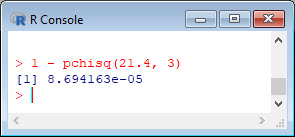Let us apply Hosmer and Lemeshow goodness of fit (GOF) test.
library(ResourceSelection)
hoslem.test(mtcars\$vs, fitted(model))Unfortunately, we must reject the null hypothesis (i.e. our model) because the fitted values are significantly different from the data.
plot(sort(model\$fitted), pch = 16, cex.lab = 1.3, ylim = c(0, 1))
points(sort(mtcars\$vs), pch = 19, col = "red", cex = 1.3)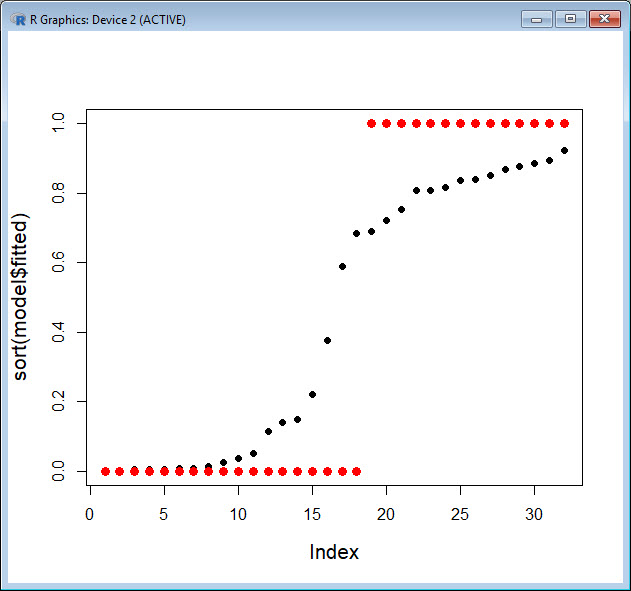Let’s try another plot, this time joining the fitted values with straight lines.
plot(sort(mtcars\$vs), pch = 19, col = "red", cex = 1.3, ylab = "Proportion")
points(sort(model\$fitted), ty = "o", pch = 16, cex.lab = 1.3, ylim = c(0, 1))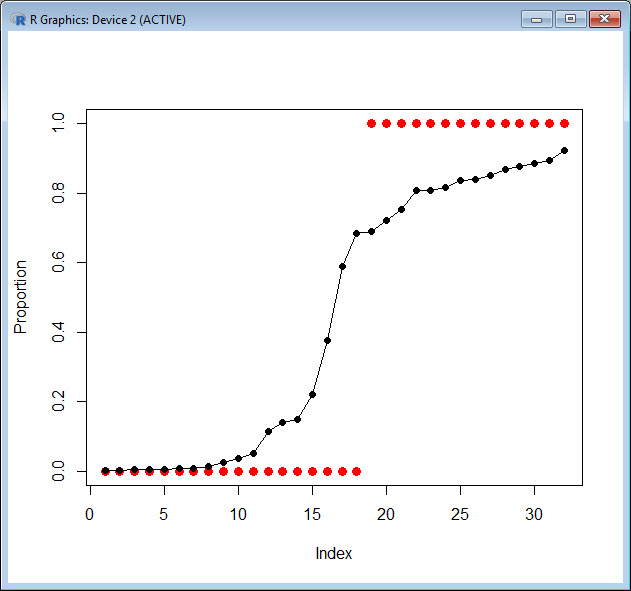The glm() has produced a kind of density that relates the observations for vs = 0 to those for vs = 1.
We may wish to plot for each predictor separately, so first we fit a glm for weight.
model_weight <- glm(formula = vs ~ wt, data = mtcars, family = binomial) summary(model_weight)range(mtcars\$wt)xweight <- seq(0, 6, 0.01) yweight <- predict(model_weight, list(wt = xweight),type="response") plot(mtcars\$wt, mtcars\$vs, pch = 16, xlab = "WEIGHT (g)", ylab = "VS") lines(xweight, yweight)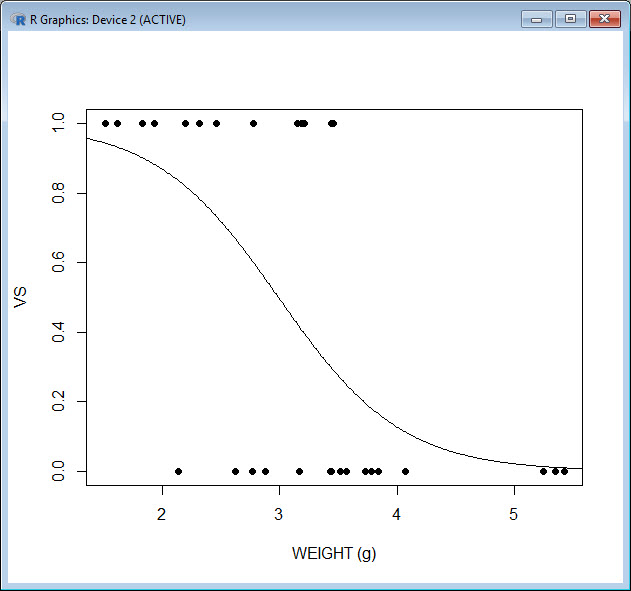model_disp <- glm(formula = vs ~ disp, data = mtcars, family = binomial) summary(model_disp)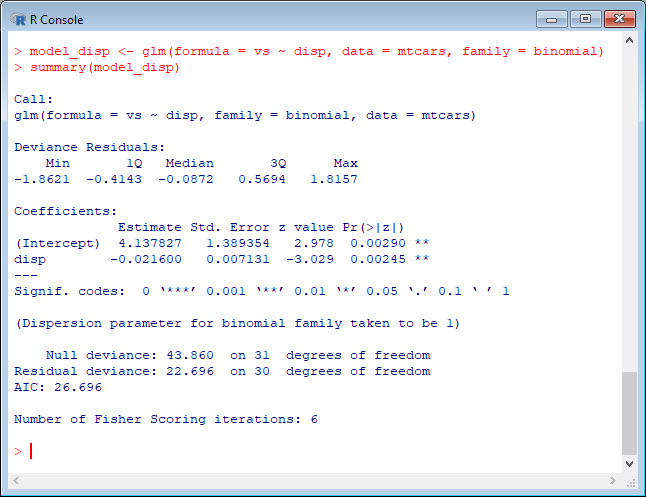range(mtcars\$disp)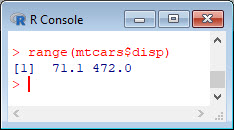xdisp <- seq(0, 500, 0.01) ydisp <- predict(model_disp, list(disp = xdisp),type="response") plot(mtcars\$disp, mtcars\$vs, pch = 16, xlab = "DISPLACEMENT (cubic inches)", ylab = "VS") lines(xdisp, ydisp)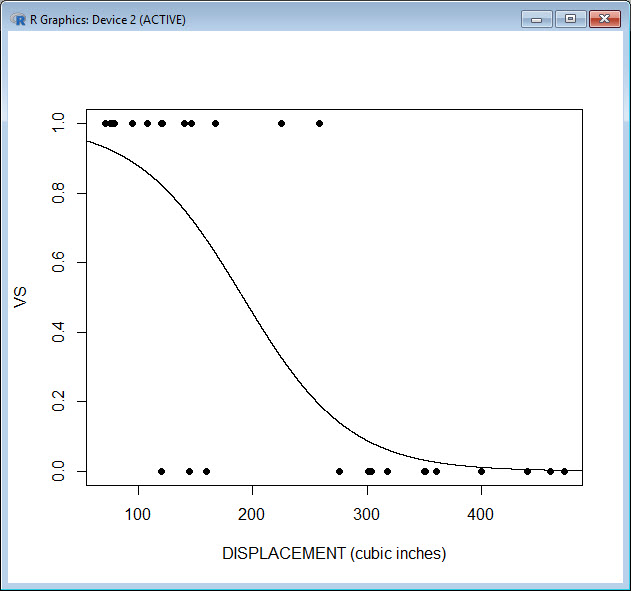That wasn’t so hard! In Blog 17 I will explain more about the output we got from the glm() function.
See you later!
David

Annex: R codes used

# Estimate Generalised Linear Model and display results.
model <- glm(formula = vs ~ wt + disp, data = mtcars, family = binomial)
summary(model)

# Calculate Chi-square statistic.
1 - pchisq(21.4, 3)

library(ResourceSelection)

# Apply Hosmer and Lemeshow goodness of fit (GOF) test.
hoslem.test(mtcars\$vs, fitted(model))

# Graph estimated model.
plot(sort(model\$fitted), pch = 16, cex.lab = 1.3, ylim = c(0, 1))
points(sort(mtcars\$vs), pch = 19, col = "red", cex = 1.3)

# Alternative graph of the estimated model.
plot(sort(mtcars\$vs), pch = 19, col = "red", cex = 1.3, ylab = "Proportion")
points(sort(model\$fitted), ty = "o", pch = 16, cex.lab = 1.3, ylim = c(0, 1))

# Estimate Generalised Linear Model and display results.
model_weight <- glm(formula = vs ~ wt, data = mtcars, family = binomial)
summary(model_weight)

# Calculate range of values.
range(mtcars\$wt)

# Graph estimated model.
xweight <- seq(0, 6, 0.01)
yweight <- predict(model_weight, list(wt = xweight), type = "response")
plot(mtcars\$wt, mtcars\$vs, pch = 16, xlab = "WEIGHT (g)", ylab = "VS")
lines(xweight, yweight)

# Estimate Generalised Linear Model and display results.
model_disp <- glm(formula = vs ~ disp, data = mtcars, family = binomial)
summary(model_disp)

# Graph estimated model.
xdisp <- seq(0, 500, 0.01)
ydisp <- predict(model_disp, list(disp = xdisp), type = "response")
plot(mtcars\$disp, mtcars\$vs, pch = 16, xlab = "DISPLACEMENT (cubic inches)", ylab = "VS")
lines(xdisp, ydisp)